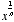nth root

nth Root

The number that must be multiplied times itself n times to equal a given value. The nth root of x is writtenor. For example,since 25 = 32.

Notes: When n = 2 an nth root is called a square root. Also, if n is even and x is negative, thenis nonreal.